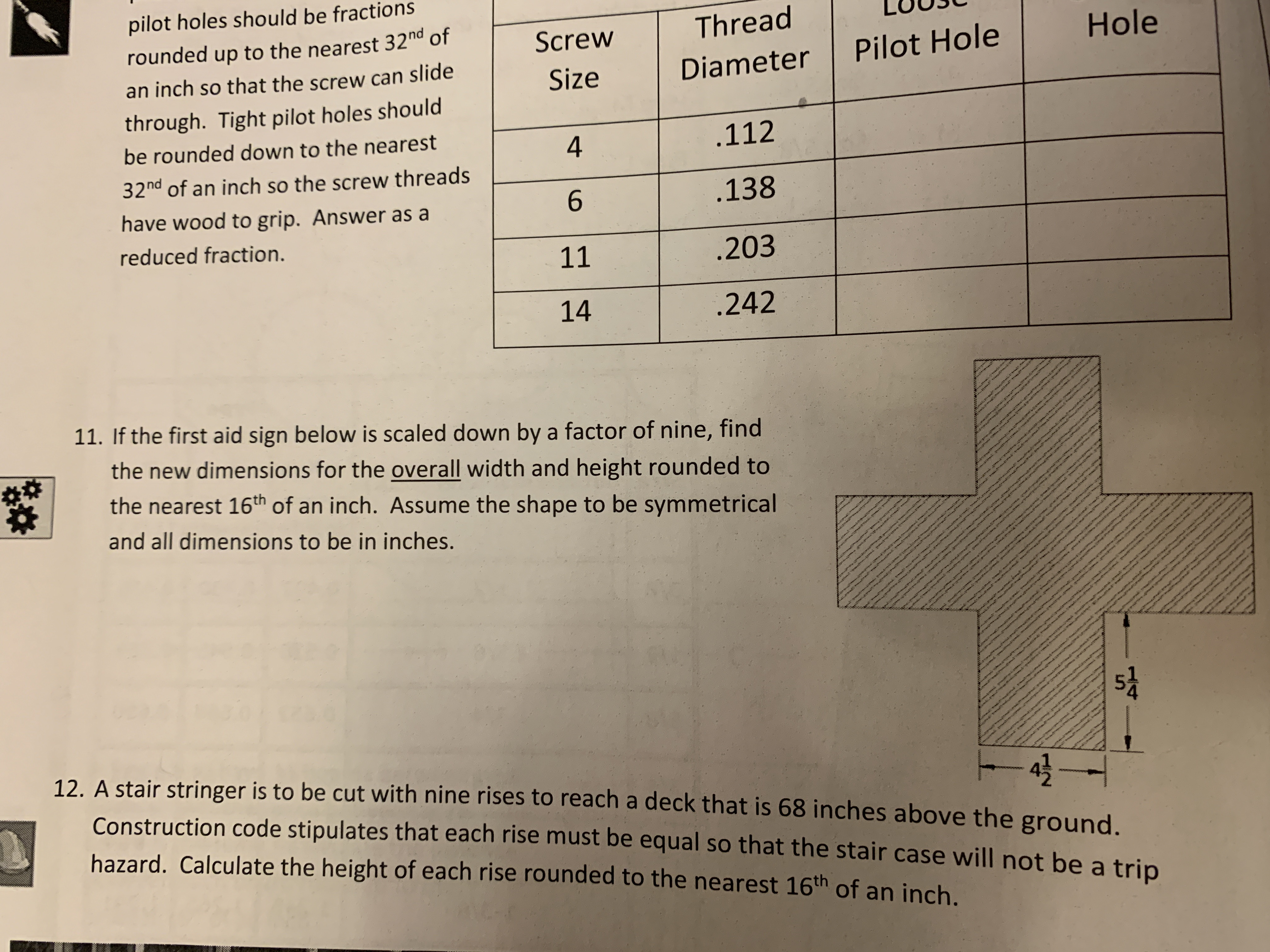# pilot holes should be fractions rounded up to the nearest 32nd of an inch so that the screw can slide through. Tight pilot holes should be rounded down to the nearest 32nd of an inch so the screw threads have wood to grip. Answer as a reduced fraction Screw Thread LUU3L ThreadUUL Size Diameter Pilot Hole Hole 112 .138 11203 14 .242 11. If the first aid sign below is scaled down by a factor of nine, find the new dimensions for the overall width and height rounded to the nearest 16th of an inch. Assume the shape to be symmetrical and all dimensions to be in inches. At 1 5 12. A stair stringer is to be cut with nine rises to reach a deck that is 68 inches above the ground. 42 Construction code stipulates that each rise must be equal so that the stair case will not be a trip hazard. Calculate the height of each rise rounded to the nearest 16th of an inch.

Question

Number 11.help_outlineImage Transcriptionclosepilot holes should be fractions rounded up to the nearest 32nd of an inch so that the screw can slide through. Tight pilot holes should be rounded down to the nearest 32nd of an inch so the screw threads have wood to grip. Answer as a reduced fraction Screw Thread LUU3L ThreadUUL Size Diameter Pilot Hole Hole 112 .138 11203 14 .242 11. If the first aid sign below is scaled down by a factor of nine, find the new dimensions for the overall width and height rounded to the nearest 16th of an inch. Assume the shape to be symmetrical and all dimensions to be in inches. At 1 5 12. A stair stringer is to be cut with nine rises to reach a deck that is 68 inches above the ground. 42 Construction code stipulates that each rise must be equal so that the stair case will not be a trip hazard. Calculate the height of each rise rounded to the nearest 16th of an inch. fullscreen